IMO Shortlist 2011 problem N7

Kvaliteta:
Avg: 0,0
Težina:
Avg: 9,0
Let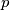$p$ be an odd prime number. For every integer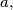$a,$ define the number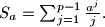$S_a = \sum^{p-1}_{j=1} \frac{a^j}{j}.$ Let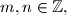$m,n \in \mathbb{Z},$ such that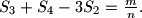$S_3 + S_4 - 3S_2 = \frac{m}{n}.$ Prove that$p$ divides$m.$

Proposed by Romeo Meštrović, Montenegro
Izvor: Međunarodna matematička olimpijada, shortlist 2011